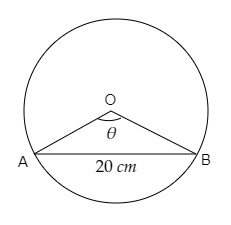# In a circle of diameter $40 \mathrm{~cm}$ the length of a chord is $20 \mathrm{~cm}$. Find the length of minor arc corresponding to the chord.

Given:

In a circle of diameter $40 \mathrm{~cm}$ the length of a chord is $20 \mathrm{~cm}$.

To do:

We have to find the length of the minor arc corresponding to the chord.

Solution:The diameter of the circle $=40\ cm$

Radius (r) of the circle $= \frac{40}{2}\ cm=20\ cm$

Let AB be a chord of the circle.

In $\triangle OAB, OA = OB = r = 20\ cm$

$AB = 20\ cm$

This implies,

$\triangle OAB$ is an equilateral triangle.

$\theta=60^o=\frac{\pi}{3}\ cm$

We know that in a circle of radius r unit, if an arc of length $l$ unit subtends an angle $\theta$ radian at the centre, then $\theta= \frac{l}{r}$

$\frac{\pi}{3}=\frac{AB}{20}$

$AB=\frac{20\pi}{3}\ cm$

The length of the minor arc is $\frac{20\pi}{3}\ cm$.

Updated on: 10-Oct-2022

30 Views